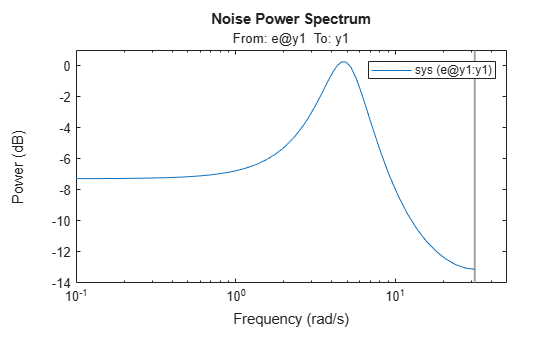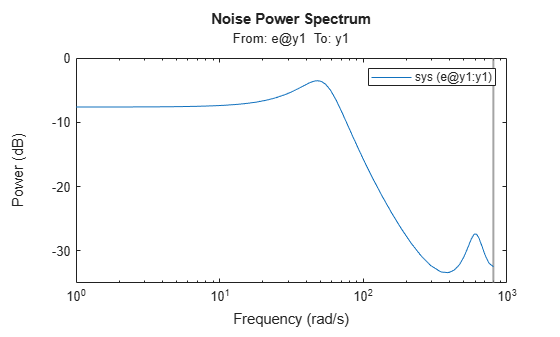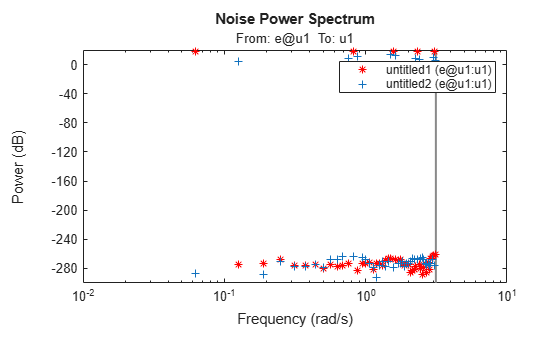# spectrum

Output power spectrum of time series models

## Syntax

```spectrum(sys) spectrum(sys,{wmin, wmax}) spectrum(sys,w) spectrum(sys1,...,sysN,w) ps = spectrum(sys,w) [ps,w] = spectrum(sys) [ps,w,sdps] = spectrum(sys) ```

## Description

`spectrum(sys)` creates an output power spectrum plot of the identified time series model `sys`. The frequency range and number of points are chosen automatically.

`sys` is a time series model, which represents the system:

`$y\left(t\right)=He\left(t\right)$`

Where, `e(t)` is a Gaussian white noise and `y(t)` is the observed output.

`spectrum` plots `abs(H'H)`, scaled by the variance of `e(t)` and the sample time.

If `sys` is an input-output model, it represents the system:

`$y\left(t\right)=Gu\left(t\right)+He\left(t\right)$`

Where, `u(t)` is the measured input, `e(t)` is a Gaussian white noise and `y(t)` is the observed output.

In this case, `spectrum` plots the spectrum of the disturbance component `He(t)`.

`spectrum(sys,{wmin, wmax})` creates a spectrum plot for frequencies ranging from `wmin` to `wmax`.

`spectrum(sys,w)` creates a spectrum plot using the frequencies specified in the vector `w`.

`spectrum(sys1,...,sysN,w)` creates a spectrum plot of several identified models on a single plot. The `w` argument is optional.

You can specify a color, line style and marker for each model. For example:

`spectrum(sys1,'r',sys2,'y--',sys3,'gx');`

`ps = spectrum(sys,w)` returns the power spectrum amplitude of `sys` for the specified frequencies, `w`. No plot is drawn on the screen.

`[ps,w] = spectrum(sys)` returns the frequency vector, `w`, for which the output power spectrum is plotted.

```[ps,w,sdps] = spectrum(sys)``` returns the estimated standard deviations of the power spectrum.

For discrete-time models with sample time `Ts`, `spectrum` uses the transformation `z = exp(j*w*Ts)` to map the unit circle to the real frequency axis. The spectrum is only plotted for frequencies smaller than the Nyquist frequency `pi/Ts`, and the default value 1 (time unit) is assumed when Ts is unspecified.

## Input Arguments

 `sys` Identified model. If `sys` is a time series model, it represents the system: `$y\left(t\right)=He\left(t\right)$` Where, `e(t)` is a Gaussian white noise and `y(t)` is the observed output. If `sys` is an input-output model, it represents the system: `$y\left(t\right)=Gu\left(t\right)+He\left(t\right)$` Where, `u(t)` is the measured input, `e(t)` is a Gaussian white noise and `y(t)` is the observed output. `wmin` Minimum frequency of the frequency range for which the output power spectrum is plotted. Specify `wmin` in `rad/TimeUnit`, where `TimeUnit` is `sys.TimeUnit`. `wmax` Maximum frequency of the frequency range for which the output power spectrum is plotted. Specify `wmax` in `rad/TimeUnit`, where `TimeUnit` is `sys.TimeUnit`. `w` Frequencies for which the output power spectrum is plotted. Specify `w` in `rad/TimeUnit`, where `TimeUnit` is `sys.TimeUnit`. `sys1,...,sysN` Identified systems for which the output power spectrum is plotted.

## Output Arguments

 `ps` Power spectrum amplitude. If `sys` has `Ny` outputs, then `ps` is an array of size `[Ny Ny length(w)]`. Where `ps(:,:,k)` corresponds to the power spectrum for the frequency at `w(k)`. For amplitude values in dB, type `psdb = 10*log10(ps)`. `w` Frequency vector for which the output power spectrum is plotted. `sdps` Estimated standard deviation of the power spectrum.

## Examples

collapse all

`load iddata1 z1;`

Estimate a single-input single-output state-space model.

`sys = n4sid(z1,2);`

Plot the noise spectrum for the model.

`spectrum(sys);``load iddata9 z9`

Estimate a fourth-order AR model using a least-squares approach.

`sys = ar(z9,4,'ls');`

Plot the output spectrum of the model.

`spectrum(sys);`Create an input consisting of five sinusoids spread over the whole frequency interval. Compare the spectrum of this signal with that of its square. The frequency splitting (the square having spectral support at other frequencies) reveals the nonlinearity involved.

```u = idinput([100 1 20],'sine',[],[],[5 10 1]); u = iddata([],u,1,'per',100); u2 = u.u.^2; u2 = iddata([],u2,1,'per',100); spectrum(etfe(u),'r*',etfe(u2),'+')```